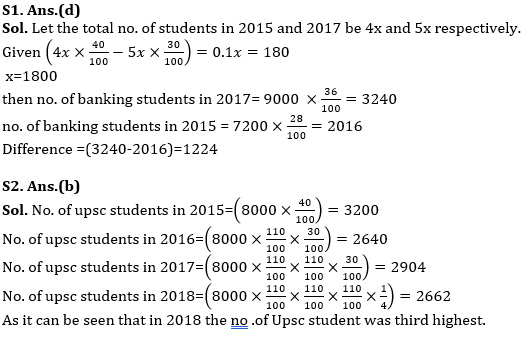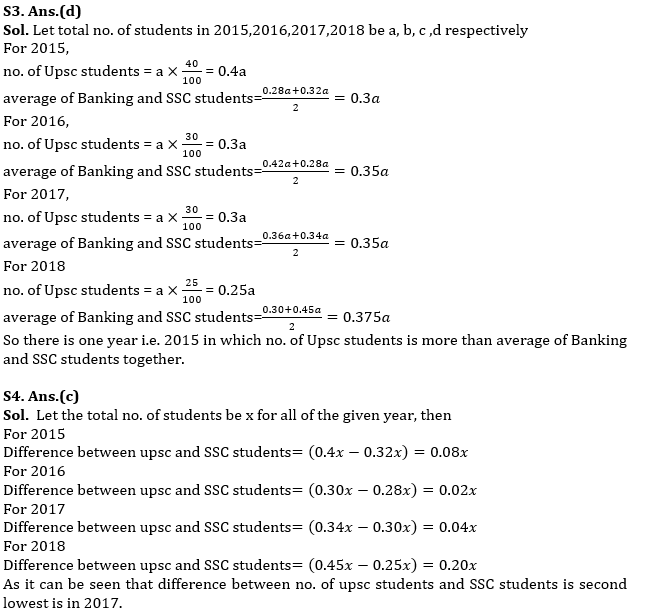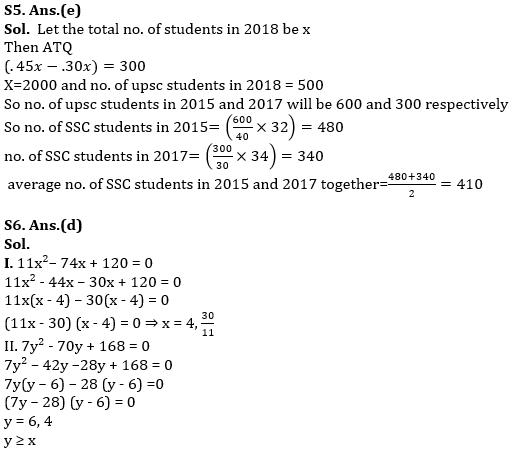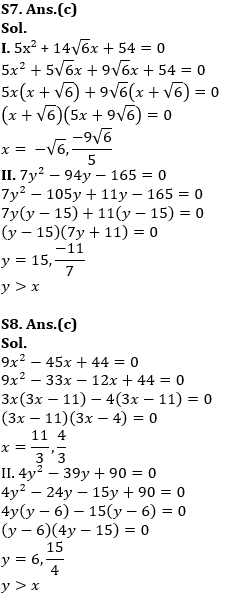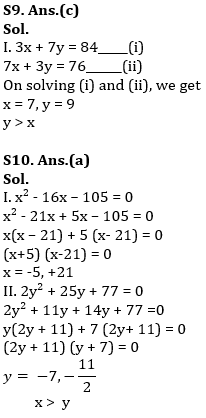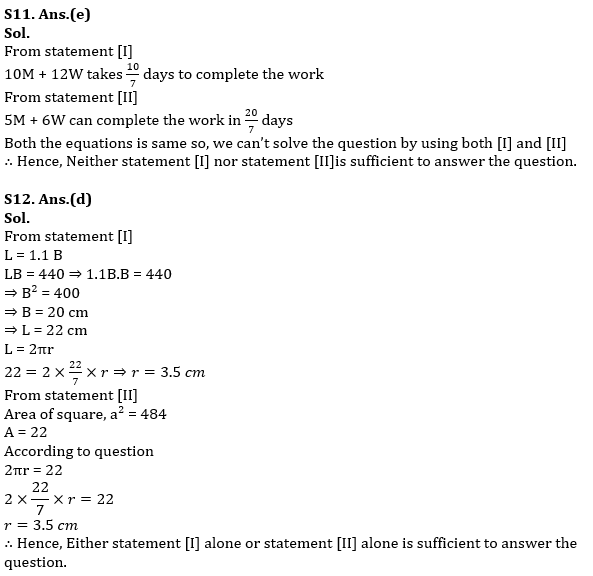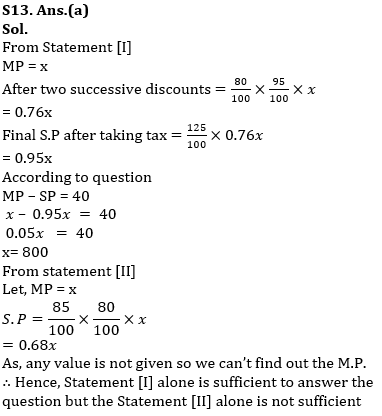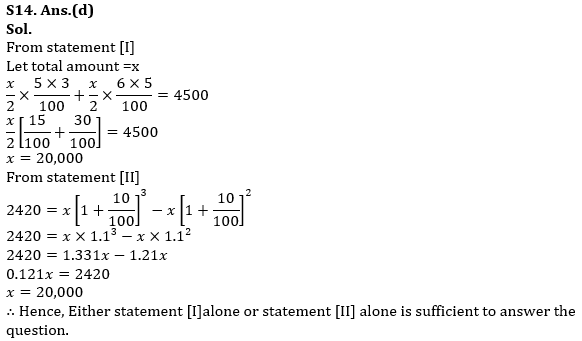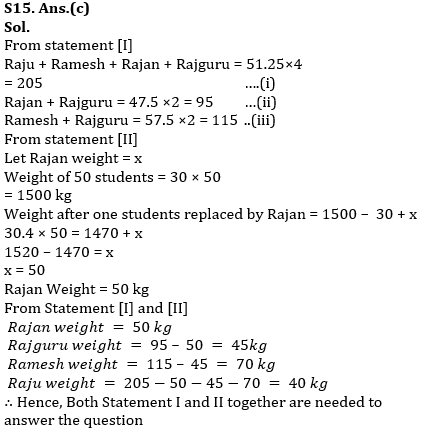Latest Banking jobs   »   Quantitative Aptitude Quiz For RBI Grade...

# Quantitative Aptitude Quiz For RBI Grade B/ ECGC PO/ SIDBI Grade A Prelims 2022- 17th April

Direction (1-5): The Bar graph shows the no. of Students (in %) enrolled in two different courses out of three different courses for four different years of ”Career Power” coaching. Study the graph carefully to answer the following questions.
Total no. of Student=(SSC + Banking +Upsc) Students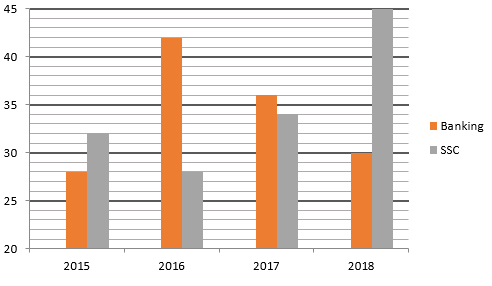Q1. If total student in 2015 & 2017 are in ratio of 4:5 and the difference between no. of Upsc students in these two years is 180 then find the difference between no. of Banking students in these two years?
(a) 1218
(b) 1332
(c) 1418
(d) 1224
(e) 1350

Q2.If total student in 2015 is 8000 and increased at 10% annually for the following years then find in which year the no. of Upsc student was third highest?
(a) 2016
(b) 2018
(c) 2017
(d) none of these
(e) can’t be determined

Q3.For how many year the no. of Upsc student is more than the average of the no. of student of the rest two courses?
(a) 0
(b) 2
(c) 3
(d) 1
(e) None of these

Q4.If the total no. of students is constant for all the years then find in which year the difference between no. of upsc students and SSC students is second lowest?
(a) 2015
(b) 2016
(c) 2017
(d) 2018
(e) none of these

Q5.If the ratio of upsc students in 2015,2017,2018 be 6:3:5 and difference between Banking and SSC students in 2018 Is 300, then find the average no. of SSC students in 2015 and 2017 together?
(a) 590
(b) 640
(c) 240
(d) 190
(e) 410

Directions (6-10): In the following questions two equations numbered (I) and (II) are given. You have to solve both equations and give answer
(a) if x > y
(b) if x ≥ y
(c) if x < y
(d) if x ≤ y
(e) If x = y or the relationship cannot be established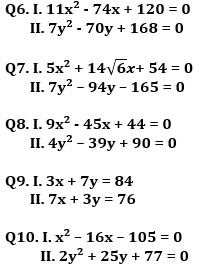Directions (11-15): Each question below is followed by two Statements [I] and [II]. You have to determine whether the data given in the statements are sufficient for answering the question. You should use the data and your knowledge of Mathematics to choose between the possible answers.
(a) if the Statement [I] alone is sufficient to answer the question but the Statement [II] alone is not sufficient
(b) if the Statement [II] alone is sufficient to answer the question but the Statement [I] alone is not sufficient
(c) if both Statement [I] and [II] together are needed to answer the question
(d) if either the Statement [I] alone or Statement [II] alone is sufficient to answer the question
(e) if you cannot get the answer from the Statements [I] and [II] together but need even more data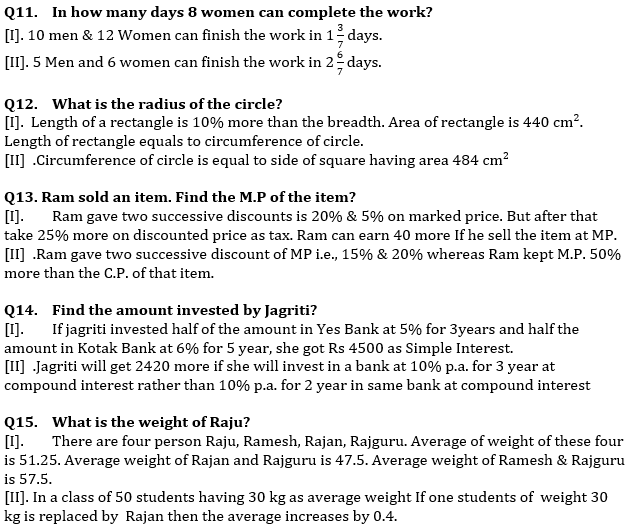Solutions